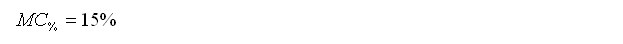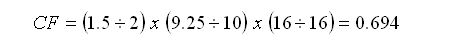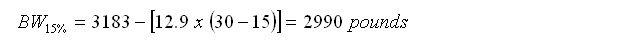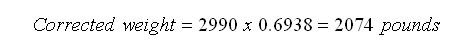# Calculating the weight of lumber

Formulas, detailed instructions and species-specific charts from Professor Gene Wengert. May 3, 2001

by Professor Eugene Wengert

The average weight of 1000 board feet (BF) of lumber can be calculated for a particular species, by following the procedures below. Be aware though, there is considerable variation from load to load of the same species.

Step 1:
Determine the moisture content (MC) at which the lumber was scaled (that is, at which the board foot volume was measured). In the weight calculation equations, this value is calledRemember that moisture content is calculated with the following equation: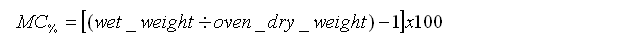Step 2:
Determine the correction factor (CF) that must be applied to the scaled volume to determine the actual volume of wood. Use the following formula:

 CF = (actual thickness in inches � nominal thickness in inches) x (actual width in inches � nominal width in inches) x (actual length in feet � nominal length in feet)

CF Example 1:
A load of green (freshly sawn) hardwood lumber is 1-1/8" inches thick, but is counted as 1 inch thick when scaled for board footage. The lumber is in random widths, for which the actual and scaled widths are equal. All lumber is 98 inches long (or 8.17 feet), and is scaled as 8 foot. So,

 CF = (1.125 � 1) x (actual width � scaled width) x (8.17 � 8) = 1.125 x 1 x 1.021 = 1.149

CF Example 2:
The same scenario as in Example 1, except the lumber is 2-5/32 inches thick (scaled as 2 inches thick) and exactly 14 feet in length. The lumber is again in random widths. So,

 CF = (2.156 � 2) x (actual width � scaled width) x (14 � 14) = 1.078 x 1 x 1 = 1.078

CF Example 3:
Planed softwood lumber is scaled as two-by-sixes, although the actual thickness is 1.5 inches and the actual width is 5.5 inches. The two-by-sixes are exactly 12 feet long. Therefore,

 CF = (1.5 � 2) x (5.5 � 6) x (12 � 12) = 0.75 x 0.917 x 1 = 0.688

Step 3:
Determine the basic weight forof the desired species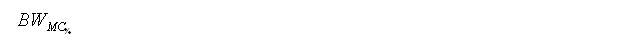using Table 1 and one of the following formulas: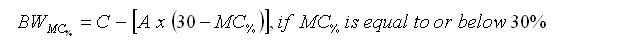Step 4:
Apply the board foot correction factor, CF, from equation 2, to arrive at the weight, in pounds, per 1000 BF of lumber, corrected for scaling errors and moisture content:Step 5:
If the weight of the same lumber at a different moisture contentis desired, use the following formula:Weight Calculation Examples

Example 1:
What is the weight of 1000 BF of 75% MC, 4/4 northern red oak (scaled thickness = 1 inch), 1-1/8 inches thick and 12 feet long?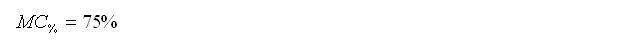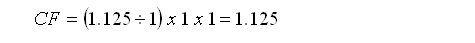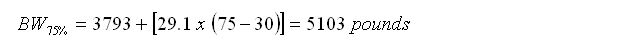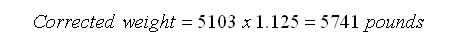Example 2:
The lumber in Example 1 is subsequently dried to 6% MC. What is the new weight?Note: This lumber has shrunk about 6% in going from 75% MC to 6% MC, so it is no longer 1000 BF at 6% MC, but about 940 BF. Yet, because it was 1000 BF when measured green, we base the calculations on the green size and weight. If it were rescaled at 6% MC, the calculations would be redone.

Example 3:
What is the weight of 1000 BF of northern red oak that was cut to 1-5/8 inches (1.625") when green, but, when scaled as 6/4 lumber (1-1/2 inches thick) at 6% MC, had actually shrunk to 1-9/16 inches thick (1.5625")?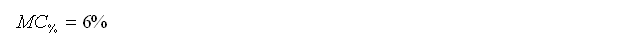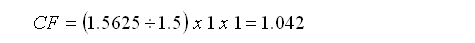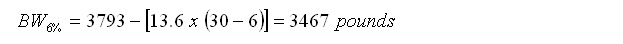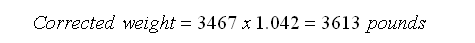Example 4:
What is the weight of 1000 BF of loblolly pine 2" x 10" x 16' lumber (acual size: 1.5" x 9.25" x 16') at 15% MC? The footage is measured at 15% MC.